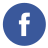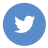### fibonacci series in java

java

The Fibonacci series is a sequence of numbers in which each number is the sum of the previous two numbers. The series starts with 0 and 1, and the subsequent numbers are calculated by adding the previous two. For example, the Fibonacci series is: 0, 1, 1, 2, 3, 5, 8, 13, 21, 34, and so on.

Here is an example of how you can write a Java program to generate the Fibonacci series:

``````public class Fibonacci {
public static void main(String[] args) {
int n = 10; // number of terms to generate
int a = 0, b = 1, c; // a and b are the first two terms, c is the next term
System.out.print(a + " " + b + " "); // print the first two terms
for (int i = 2; i < n; i++) { // start the loop from the third term
c = a + b; // calculate the next term
System.out.print(c + " "); // print the term
a = b; // update the value of a
b = c; // update the value of b
}
}
}``````

This program will output the first 10 terms of the Fibonacci series: 0 1 1 2 3 5 8 13 21 34.

Note: This program uses a loop to generate the terms of the series. The loop starts from the third term (i = 2) and continues until it reaches the nth term (i < n). At each iteration, the next term is calculated by adding the previous two terms (c = a + b), and the values of a and b are updated to the value of b and c, respectively.